## Unity and Phi^3

Discussion concerning the first major re-evaluation of Dewey B. Larson's Reciprocal System of theory, updated to include counterspace (Etheric spaces), projective geometry, and the non-local aspects of time/space.
ckiit
Posts: 30
Joined: Sat Dec 21, 2019 7:54 am

### Unity and Phi^3

In attempt to produce a universal "field equation" based on the known transcendental universal phenomena
of phi (ϕ, as a scalar linear "base") and pi (π, as a rotating "base") I discovered something extremely important
as it may relate to the framework of RS/2 utilizing '1' as unity:
because squaring π+π√5/2π (golden ratio 1.618...) introduces '1' to produce 2.618...,
if it is somehow possible to assign this particular 1 to/as the the universal datum,
a universal geometry can be derived which naturally "feeds off" of the phi/pi operation ad infinitum.
This geometry would naturally produce the forms associated with the golden ratio. I prove this is true
with the following image generated with the derived equation (cos):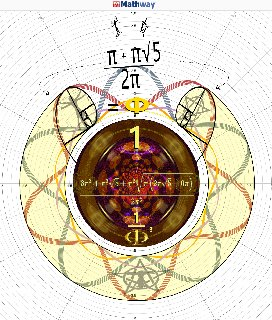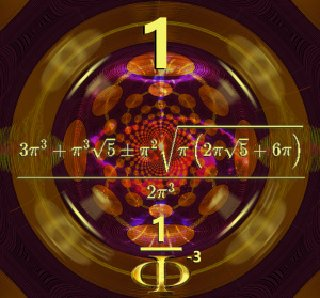These were generated by a generic online mathematics website with a simple sin function applied to the concerned equation.

I started thus with these as co-operative bases: one linear (phi), the other rotating (pi).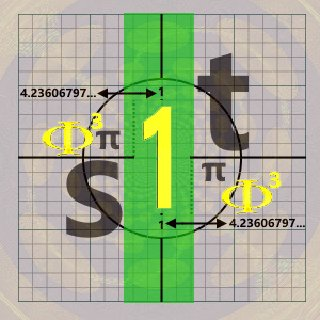Essentially these equations capture a unity-golden framework:
one state (-) is unity 1, the other (+) is ϕ3.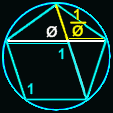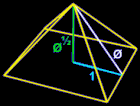This is important because it confirms the bi-rotation model (mandated by the geometry as intrinsic)
and implies a bi-orientation compliment, the properties of which can be derived inductively -
the implications of which amounts to the capacity to calculate universal roots using universal geometries
that satisfy cubed proportionality (as time and space reciprocally do).

user737
Posts: 74
Joined: Wed Oct 24, 2018 7:39 pm

### Re: Unity and Phi^3

It might be of interest to you to review the attached file for my thoughts on the matter.

Of note, it looks as though there is a mathematical corollary to the geometric concept of dimensional reduction/expansion with regard to phi

Φ = (1 + √5)/2
Attachmentsplaying_with_phi.xlsx
Infinite Rider on the Big Dogma

ckiit
Posts: 30
Joined: Sat Dec 21, 2019 7:54 am

### Re: Unity and Phi^3

user737 wrote:
Tue Jan 28, 2020 8:42 pm
It might be of interest to you to review the attached file for my thoughts on the matter.

Of note, it looks as though there is a mathematical corollary to the geometric concept of dimensional reduction/expansion with regard to phi

Φ = (1 + √5)/2
Thanks, this is great: unfortunately I can not open office files on this computer, but will take a look when possible.

What are the odds of a single identity whose +/- root solutions are +1 and +phi^3, thus scale invariant?
Would that not be an equation which describes the physical universe?

user737
Posts: 74
Joined: Wed Oct 24, 2018 7:39 pm

### Re: Unity and Phi^3

ckiit wrote:
Wed Jan 29, 2020 3:55 am
What are the odds of a single polynomial whose +/- root solutions are +1 and +phi^3, thus scale invariant?
Would that not be an equation which describes the physical universe?
3 = Φ2 + Φ = Φ2 / (Φ-1) = (Φ+1) / (Φ-1) = -Φ × Φ2= -Φ3

(1/Φ)2 + 1/Φ = +1

where Φ = (1 + √5)/2 -- the golden ratio
Infinite Rider on the Big Dogma

ckiit
Posts: 30
Joined: Sat Dec 21, 2019 7:54 am

### Re: Unity and Phi^3

user737 wrote:
Wed Jan 29, 2020 2:46 pm
ckiit wrote:
Wed Jan 29, 2020 3:55 am
What are the odds of a single polynomial whose +/- root solutions are +1 and +phi^3, thus scale invariant?
Would that not be an equation which describes the physical universe?
Φ3 = Φ2 + Φ = Φ2 / (Φ-1) = (Φ+1) / (Φ-1) = -Φ3

(1/Φ)2 + 1/Φ = 1
But (1/Φ)2 + 1/Φ
≠ 1, 4.23606797...
must equal both simultaneously.

user737
Posts: 74
Joined: Wed Oct 24, 2018 7:39 pm

### Re: Unity and Phi^3

ckiit wrote:
Wed Jan 29, 2020 3:05 pm
But (1/Φ)2 + 1/Φ
≠ 1, 4.23606797...
must equal both simultaneously.
Must be missing the "imaginary" root... missing the spin ("yin") component.
Rotational (π) component is not considered, only linear ("yang").

Must use quantum π or yin π.

yang/diagrammatic π = 3.14159...

yin/kinematic/quantum π = 4

One electric displacement is 8 natural units and one magnetic displacement is 4 natural units (as magnetic:electric is 2:1)

[(Φ2 + Φ)][(1/Φ)2 + (1/Φ)] = 1 + (1/Φ) + Φ + 1

2 + Φ + (1/Φ) = 4.236067977 (Φ3)

Also where Φ = +1 the result is 4 or π

Checking work...
Infinite Rider on the Big Dogma

ckiit
Posts: 30
Joined: Sat Dec 21, 2019 7:54 am

### Re: Unity and Phi^3

user737 wrote:
Wed Jan 29, 2020 3:15 pm
ckiit wrote:
Wed Jan 29, 2020 3:05 pm
But (1/Φ)2 + 1/Φ
≠ 1, 4.23606797...
must equal both simultaneously.
Must be missing the "imaginary" root... missing the spin ("yin") component.
Rotational (π) component is not considered, only linear ("yang").

Must use quantum π or yin π.

yang/diagrammatic π = 3.14159...

yin/kinematic/quantum π = 4

One electric displacement is 8 natural units and one magnetic displacement is 4 natural units (as magnetic:electric is 2:1)

[(Φ2 + Φ)][(1/Φ)2 + (1/Φ)] = 1 + (1/Φ) + Φ + 1

2 + Φ + (1/Φ) = 4.236067977 (Φ3)

Also where Φ = +1 the result is 4 or π

Checking work...
Both solutions are "sitting" on coefficient π2:
when setting to ^1 = pie
when setting ^2 = phi
they are joined.user737
Posts: 74
Joined: Wed Oct 24, 2018 7:39 pm

### Re: Unity and Phi^3

Go ask Plato what he thinks about 1-1-Phi-1/Phi (as we have derived herein)
Infinite Rider on the Big Dogma

ckiit
Posts: 30
Joined: Sat Dec 21, 2019 7:54 am

### Re: Unity and Phi^3

user737 wrote:
Wed Jan 29, 2020 3:15 pm

yin/kinematic/quantum π = 4

One electric displacement is 8 natural units and one magnetic displacement is 4 natural units (as magnetic:electric is 2:1)

[(Φ2 + Φ)][(1/Φ)2 + (1/Φ)] = 1 + (1/Φ) + Φ + 1

2 + Φ + (1/Φ) = 4.236067977 (Φ3)

Also where Φ = +1 the result is 4 or π

Checking work...
phi of 16 = 8x2
pi of 16 = 4x4

Does the value of pi modify to 4 if Φ = +1?

ckiit
Posts: 30
Joined: Sat Dec 21, 2019 7:54 am

### Re: Unity and Phi^3

I have recovered the whole number radical expression whose inverse identities are one another: 1 and Φ³

This whole number radical expression (which is also a psuedo-quadratic) relates to the Reimann Hypothesis in that
it proves that all non-trivial zeros have nowhere else to go but the 1/2 line, therefor the algorithm which determines
the distribution of primes is attainable. I'd like to prove this formally, but because there is a prize for the solution
I will only provide the identity directly to Bruce. This identity is the real value of '1' in relation to both: Φ and π
all in a single whole number radical expression whose multiplicative inverse is Φ³. Therefor, Φ³/1 and 1/Φ³
is where the real number system begins/ends (ie. as like an ∞ fabric) whose "sum" is -1/12.

The reason for this strange sum relates to the numerator/denominator
progressively collapsing from bases 180→90→45→36→18→*16→*12
whose symmetric "pattern" is a consistent 9→9→9→9→9→*7→*3
ending with 12π/144π:
11/12 → 12/12 = 1/12
13/12 → 12/12 = -1/12
11/12 → (+) 1/12 → ***!1!*** ← 1/12 (-) ← 13/12
therefor the actual "sum" of -1/12 is an artifact, but this artifact is pointing to something extremely important:
because the real number system is enclosed, the identity of the whole radical psuedo-quadratic "encodes" information about the primes
such that the whole number '1' in relation to Φ and π is the "blueprint" of all possible primes. This would predict that the identity
must always remain '1' regardless of how many √ operations are performed on it. This is confirmed true for the concerned identity,
thus all primes must certainly fall on the 1/2 line given every single "real" number can be expressed in terms of the derived identity.

How this relates to RS2: this identity contains the universal datum of '1' and the universal counter-part Φ³, thus
by subjecting all RS2 mathematical frameworks to this identity, RS2 will "calibrate" to the real universal datum
and the related degrees of unity/disunity c=±1. This should be extremely easy to test and confirm.

I am very sorry to hear of Bruce's health issues - I hope his health endures to find this,
I know it will make RS2 stronger, if not a new 'theory of everything' inductively rooted
in the same language the universe itself obeys.